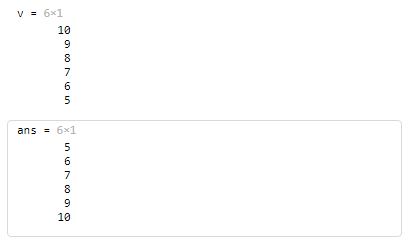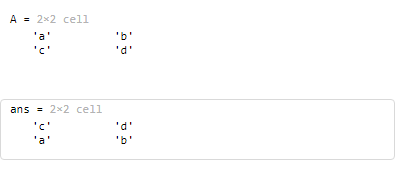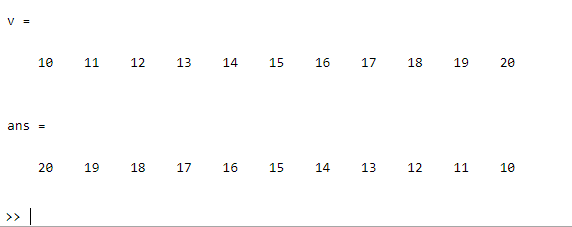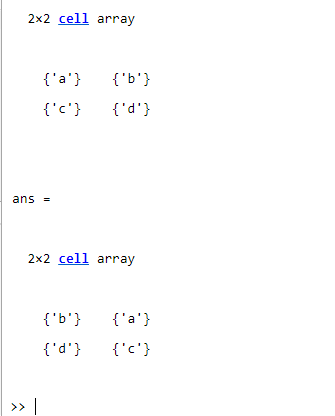Open in App
Not now

# How to inverse a vector in MATLAB?

• Last Updated : 27 Jan, 2022

In this article, we are going to discuss the “Inversion of a vector” in MATLAB which can be done in three different approaches that are illustrated below:

## Method 1: By using flipud() function

The flipud() function is used for flipping the specified vector’s elements.

Syntax:

flipud(A)

Here, flipud(A) function is used to return a vector with reversed elements of the same length of the specified vector “A”.

Example 1:

## Matlab

 `% MATLAB code for inverse a``% vector using flipud()``% Initializing a vector of some elements``v = [10;9;8;7;6;5]` `% Calling the flipud() function``% over the above vector "v" to``% reverse its elements``flipud(v)`

Output:Example 2:

## Matlab

 `% MATLAB code for inverse vector using flip()``% Initializing a 2*2 cell array of characters``A = {``'a'` `'b'``; ``'c'` `'d'``}` `% Calling the flipud() function``% over the above cell array to``% reverse its elements``flipud(A)`

Output:## Method 2: By using fliplr() function

The fliplr() function is used for flipping the specified row vector’s elements.

Syntax:’

fliplr(A)

Here, fliplr(A) function is used to return a vector with reversed elements of the same length of the specified row vector “A”.

Example 1:

## Matlab

 `% MATLAB code for fliplr()``% Initializing a row vector``v = 10:20` `% Calling the fliplr() function``% over the above vector "v" to``% reverse its elements``fliplr(v)`

Output:Example 2:

## Matlab

 `% MATLAB code for fliplr()``% Initializing a 2*2 cell``% array of characters``A = {``'a'` `'b'``; ``'c'` `'d'``}` `% Calling the fliplr() function``% over the above cell array "A" to``% reverse its elements``fliplr(A)`

Output:## Method 3: By using “vector(end:-1:1)” operation

The end keyword in MATLAB is used for  either finding a row or column index to reference the last element,

Example 1:

## Matlab

 `% MATLAB code for find inverse``% of vector using end keyword``% Initializing a vector of some elements``v = [10;9;8;7;6;5]` `% Reversing the above vector's elements``v(``end``:-1:1)`

Output:Example 2:

## Matlab

 `% MATLAB code for find inverse``% of vector using end keyword``% Initializing a row vector``v = 10:20` `% Reversing the above vector's elements``v(``end``:-1:1)`

Output:My Personal Notes arrow_drop_up